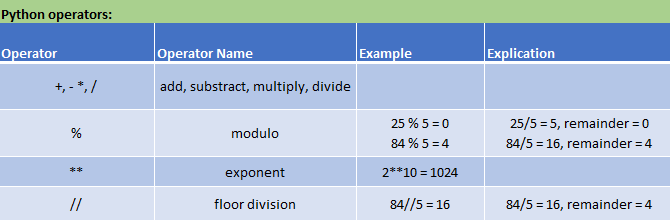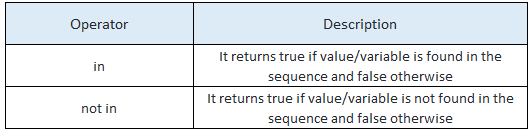### Python Operators and Expressions

Operators are special symbols that are useful for doing computations like addition, subtraction, multiplication, division, and exponentiation etc. The operators are always applied to some values which are called operands.

Python has so many built-in operators to perform different arithmetic and logical operations. There are mainly 7 types of operators in Python.

1. Arithmetic Operators
2. Relational Operators
3. Logical Operators
4. Bitwise Operators
5. Assignment operators
6. Identity operators
7. Membership operators

### Arithmetic Operators :Copy and paste the code into the below box and click the run button to see the output.

``````print(35/6)
print(3.14*10)
print(10+41)
print(10%4)
print(5**2)
(5+9)*(15-7)``````

#### Relational Operators:

Below table shows the relational operators in Python.These operators are used to compare values.``````a=10
b=10
print(a<b)
print(a>b)
print(a==b)
print(a<=b)
print(a>=b)``````

#### Bitwise Operators:

Bitwise operators act on operands bit by bit as if they are string of binary digits.For example, 1 is 01 in binary and 2 is 10. Now, apply Bitwise Operators and play with it in the below box.

```a=1
b=2
print(a&b)
print(a|b)
print(a^b)
print(~a)
print(a<<b)
print(a>>b)```

#### Assignment operators:

In Python, we use Assignment operators to assign values to variables. Following table covers all assignment operators available in Python.#### Identity operators:

There two identity operators in Python are is and is not. we use Identity Operators to compare the memory location of two objects.```a = 2
b=7
print(a is not b)
print(a is b)

x=[1,2,3]
y=[1,2,3]
print(x is y)```

#### Membership Operators:

In Python, there are operators that are mainly useful to test for membership in a sequence such as lists, strings or tuples.operators test for membership in a sequence such as lists, strings, tuples, set and dictionary.```a=[1,2,3,4]
b=3
print(b in a)

x="Make Me Analyst"
y="Analyst"

print(y in x)
print(y not in x)

```

#### Expressions:

An expression is a combination of values, variables, and operators. A value all by itself is considered an expression, and so is a variable, so the following are all legal expressions:

#### Order of operations:

If more than one operator appears in an expression, the order of evaluation depends on the rules of precedence. For mathematical operators, Python follows mathematical convention. The acronym PEMDAS is a useful way to remember the rules:

1. Parentheses have the highest precedence. It can be used to force an expression to evaluate in the order you want. Since expressions in parentheses are evaluated first, 2 * (3-1) is 4, and (1+1)**(5-2) is 8. You can also use parentheses to make an expression easier to read, as in (minute * 100) / 60, even if it doesn’t change the result.
2. Exponentiation has the next highest precedence, so 2**1+1 is 3, not 4, and 3*1**3 is 3, not 27.
3. Multiplication and Division have the same precedence, which is higher than Addition and Subtraction, which also have the same precence. So 2*3-1 is 5, not 4, and 6+4/2 is 8, not 5.
4. Operators with the same precedence are evaluated from left to right. So the expression 5-3-1 is 1, not 3, because the 5-3 happens first and thened 1 is subtracted from 2.

When you have doubt, always put parentheses in your expressions to make sure the computations are performed in the order you intend.

#### String operations:

The + operator works perfectly with strings, but keep in mind that it is not addition in the mathematical sense.Actuallly, it performs concatenation, which means joining the strings by linking them end to end. For example:

```name="Mr. X"
age="30"
s="I am "+ name + "."+ "My age is "+ age
print(s)```